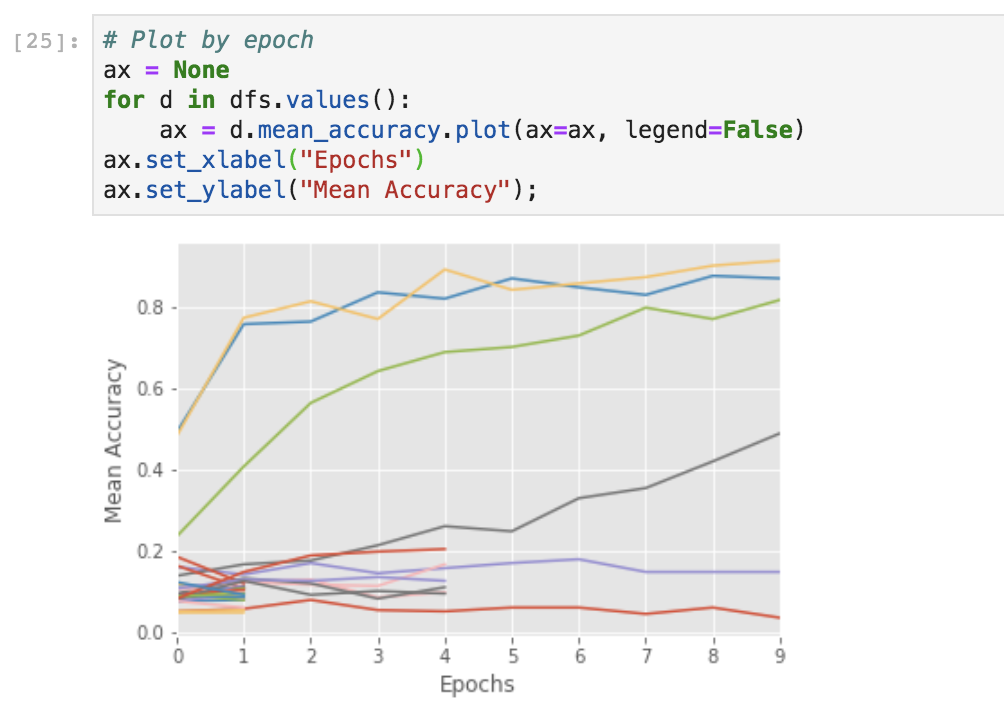# Getting Started¶

This tutorial will walk you through the process of setting up a Tune experiment. We’ll start with a PyTorch model and show you how to leverage Ray Tune to optimize the hyperparameters of this model. Specifically, we’ll leverage early stopping and Bayesian Optimization via HyperOpt to do so.

Tip

If you have suggestions on how to improve this tutorial, please let us know!

To run this example, you will need to install the following:

```\$ pip install ray torch torchvision
```

## Setting Up a Pytorch Model to Tune¶

To start off, let’s first import some dependencies. We import some PyTorch and TorchVision modules to help us create a model and train it. Also, we’ll import Ray Tune to help us optimize the model. As you can see we use a so-called scheduler, in this case the `ASHAScheduler` that we will use for tuning the model later in this tutorial.

```import numpy as np
import torch
import torch.optim as optim
import torch.nn as nn
from torchvision import datasets, transforms
import torch.nn.functional as F

from ray import tune
from ray.tune.schedulers import ASHAScheduler
```

Then, let’s define a simple PyTorch model that we’ll be training. If you’re not familiar with PyTorch, the simplest way to define a model is to implement a `nn.Module`. This requires you to set up your model with `__init__` and then implement a `forward` pass. In this example we’re using a small convolutional neural network consisting of one 2D convolutional layer, a fully connected layer, and a softmax function.

```class ConvNet(nn.Module):
def __init__(self):
super(ConvNet, self).__init__()
# In this example, we don't change the model architecture
# due to simplicity.
self.conv1 = nn.Conv2d(1, 3, kernel_size=3)
self.fc = nn.Linear(192, 10)

def forward(self, x):
x = F.relu(F.max_pool2d(self.conv1(x), 3))
x = x.view(-1, 192)
x = self.fc(x)
return F.log_softmax(x, dim=1)
```

Below, we have implemented functions for training and evaluating your Pytorch model. We define a `train` and a `test` function for that purpose. If you know how to do this, skip ahead to the next section.

## Setting up Tune¶

Below, we define a function that trains the Pytorch model for multiple epochs. This function will be executed on a separate Ray Actor (process) underneath the hood, so we need to communicate the performance of the model back to Tune (which is on the main Python process).

To do this, we call session.report in our training function, which sends the performance value back to Tune. Since the function is executed on the separate process, make sure that the function is serializable by Ray.

```def train_mnist(config):
# Data Setup
mnist_transforms = transforms.Compose(
[transforms.ToTensor(),
transforms.Normalize((0.1307, ), (0.3081, ))])

batch_size=64,
shuffle=True)
datasets.MNIST("~/data", train=False, transform=mnist_transforms),
batch_size=64,
shuffle=True)

device = torch.device("cuda" if torch.cuda.is_available() else "cpu")

model = ConvNet()
model.to(device)

optimizer = optim.SGD(
model.parameters(), lr=config["lr"], momentum=config["momentum"])
for i in range(10):

# Send the current training result back to Tune
tune.report(mean_accuracy=acc)

if i % 5 == 0:
# This saves the model to the trial directory
torch.save(model.state_dict(), "./model.pth")
```

Let’s run one trial by calling tune.run and randomly sample from a uniform distribution for learning rate and momentum.

```search_space = {
"lr": tune.sample_from(lambda spec: 10 ** (-10 * np.random.rand())),
"momentum": tune.uniform(0.1, 0.9),
}

# Uncomment this to enable distributed execution

analysis = tune.run(train_mnist, config=search_space)
```

`tune.run` returns an ExperimentAnalysis object. You can use this to plot the performance of this trial.

```dfs = analysis.trial_dataframes
[d.mean_accuracy.plot() for d in dfs.values()]
```

Note

Tune will automatically run parallel trials across all available cores/GPUs on your machine or cluster. To limit the number of concurrent trials, use the ConcurrencyLimiter.

## Early Stopping with ASHA¶

Let’s integrate early stopping into our optimization process. Let’s use ASHA, a scalable algorithm for principled early stopping.

On a high level, ASHA terminates trials that are less promising and allocates more time and resources to more promising trials. As our optimization process becomes more efficient, we can afford to increase the search space by 5x, by adjusting the parameter `num_samples`.

ASHA is implemented in Tune as a “Trial Scheduler”. These Trial Schedulers can early terminate bad trials, pause trials, clone trials, and alter hyperparameters of a running trial. See the TrialScheduler documentation for more details of available schedulers and library integrations.

```analysis = tune.run(
train_mnist,
num_samples=20,
scheduler=ASHAScheduler(metric="mean_accuracy", mode="max"),
config=search_space,
)

# Obtain a trial dataframe from all run trials of this `tune.run` call.
dfs = analysis.trial_dataframes
```

You can run the below in a Jupyter notebook to visualize trial progress.

```# Plot by epoch
ax = None  # This plots everything on the same plot
for d in dfs.values():
ax = d.mean_accuracy.plot(ax=ax, legend=False)
```You can also use TensorBoard for visualizing results.

```\$ tensorboard --logdir {logdir}
```

## Search Algorithms in Tune¶

In addition to TrialSchedulers, you can further optimize your hyperparameters by using an intelligent search technique like Bayesian Optimization. To do this, you can use a Tune Search Algorithm. Search Algorithms leverage optimization algorithms to intelligently navigate the given hyperparameter space.

Note that each library has a specific way of defining the search space.

```from hyperopt import hp
from ray.tune.search.hyperopt import HyperOptSearch

space = {
"lr": hp.loguniform("lr", 1e-10, 0.1),
"momentum": hp.uniform("momentum", 0.1, 0.9),
}

hyperopt_search = HyperOptSearch(space, metric="mean_accuracy", mode="max")

analysis = tune.run(train_mnist, num_samples=10, search_alg=hyperopt_search)

# To enable GPUs, use this instead:
# analysis = tune.run(
#     train_mnist, config=search_space, resources_per_trial={'gpu': 1})

```

Note

Tune allows you to use some search algorithms in combination with different trial schedulers. See this page for more details.

You can evaluate best trained model using the ExperimentAnalysis object to retrieve the best model:

```import os

df = analysis.results_df
logdir = analysis.get_best_logdir("mean_accuracy", mode="max")